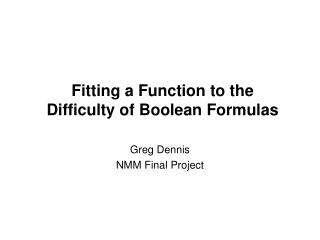DownloadDownload PresentationFitting a Function to the Difficulty of Boolean Formulas

# Fitting a Function to the Difficulty of Boolean Formulas

Télécharger la présentation## Fitting a Function to the Difficulty of Boolean Formulas

- - - - - - - - - - - - - - - - - - - - - - - - - - - E N D - - - - - - - - - - - - - - - - - - - - - - - - - - -
##### Presentation Transcript

1. Fitting a Function to theDifficulty of Boolean Formulas Greg Dennis NMM Final Project

2. Motivation • Difficulty of boolean formulas varies greatly • difficulty = # decisions by solver = time to solve • difficulty varies between formulas of same size • Difficulty depends on many factors • size of formula, clause : variable ratio, algorithm, luck • What factors influence the difficulty and to what degree? • Can we fit a function to the difficulty using these features?

3. variables literal = (negated) variable clause = disjuntion of literals CNF and 3-SAT • Conjunctive Normal Form (CNF): • k-SAT = CNF with exactly k literals / clause • no two literals in clause have same variable • no two identical clauses • k ≥ 3 is NP-complete (e.g. 3-SAT) • clausal density = # clauses / # variables CNF = conjunction of clauses

4. Phase Transition Scatter Plot

5. Phase Transition Curve

6. Unsatisfiable Core • subset of the clauses that is unsatisfiable • "proof" or "reason" for unsatisfiability • very hard to obtain a minimal core • ZChaff SAT solver iterative technique empirically close to minimal • use unsat core as feature in function fit • larger the core  more clauses solver visits

7. What I Did • examined only unsatisfiable 3-SAT formulas • generated 2550 random unsat 3-SAT • ran BerkMin SAT solver on each • ran ZChaff unsat core technique on each • recorded clauses, vars, unsat core, time • fit data with Gaussian kernel

8. time vs density

9. time vs unsat core

10. Bad Results . . . biggest outliers when unsat core = clauses

11. Better Results . . . leaving out all formulas with 100% unsatisfiable core biggest outliers when unsat core = clauses - 1

12. Observations • difficulty extremely volatile even with fixed clauses, vars, and unsat core • especially volatile at the phase transition • unsat core helps explain some difficulty, but does not tell the whole story

13. Questions Remain • curve not useful to predict the difficulty • takes longer to find unsat core than to solve • Q: could we predict unsat core if we already have the difficulty? • most applications don't generate random CNF's • Q: how well does the function predict the behavior of non-random formulas?

14. Another Experiment • performed regression again, but with time as a feature and unsat core as the value • obtained 10 CNFs generated by The Alloy Analyzer and converted them to 3-SAT • predicted the percentage of clauses in the unsat core and compared to actual number

15. Unsat Core Predictions very hard to predict . . .

16. For Future Students • lots of engineering completed • generation of random CNFs • read/write of CNFs to appropriate file format • interface with command line SAT solver • implement fix point technique • conversion to 3-SAT • regression with kernel • code, write-up, and this presentation available on my NMM page